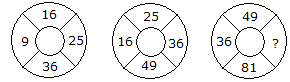# Verbal Reasoning - Character Puzzles - Discussion

### Discussion :: Character Puzzles - Character Puzzles 1 (Q.No.17)

17.

Which one will replace the question mark ?[A]. 64 [B]. 144 [C]. 169 [D]. 25

Explanation:

The numbers are squared in ascending order

In first circle,

(3)2 = 9

(4)2 = 16

(5)2 = 25

(6)2 = 36.

In second circle,

(4)2 = 16

(5)2 = 25

(6)2 = 36

(7)2 = 49.

In third circle,

(6)2 = 36

(7)2 = 49

(8)2 = 64

(9)2 = 81.

 Asadullah (Asad) said: (May 31, 2017) Another process 36 - 16 + 9 = 29 - 4 = 25. 49 - 25 + 16 = 40 - 4 = 36. 81 - 49 + 36 = 68 - 4 = 64. So the answer is 64.

 Drtey said: (Jul 8, 2020) Thanks @Asadullah

 Roshni M said: (Jan 31, 2021) Each cycle is having an difference of 2 ie, 16 - 9 = 7 25 - 16 = 9 36 - 25 = 11 49 - 36 = 13, so each answer is having a increase in 2 digit. ie 7+2=9 and so on so in the third round, we have 49 so add 49+(13+2)=49+15=64 hence answer.